Anzeige

# ch 4 gear trains.pdf

4. Feb 2023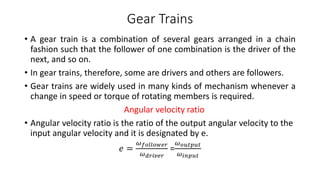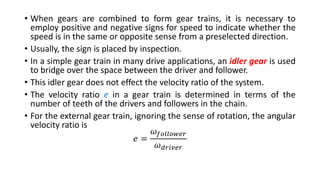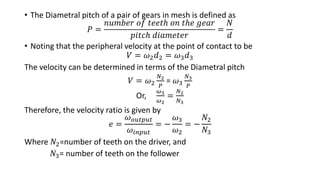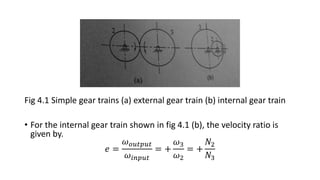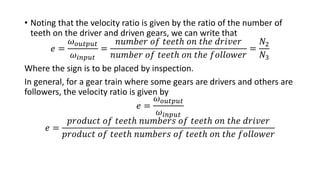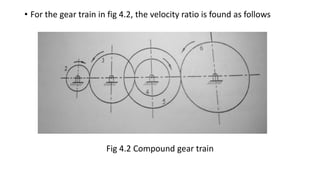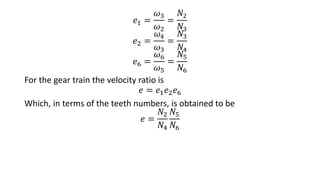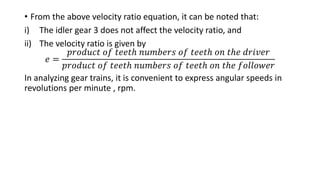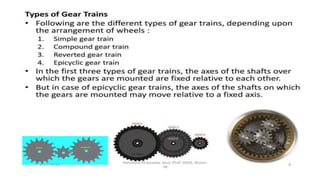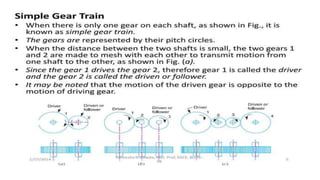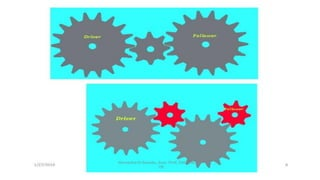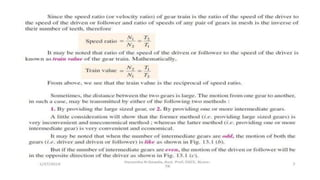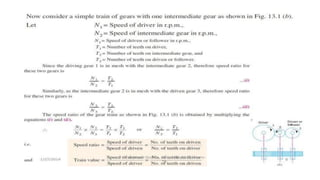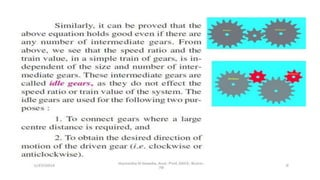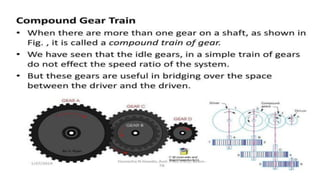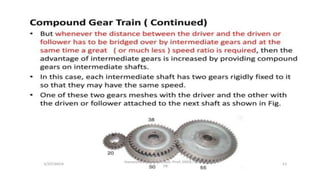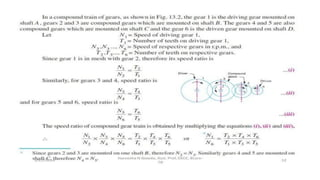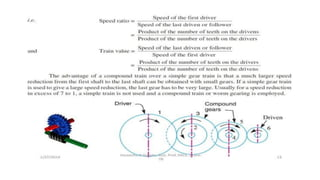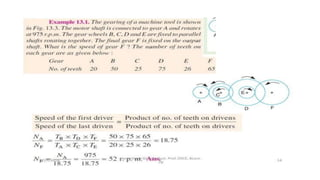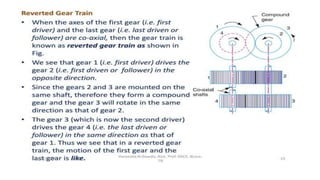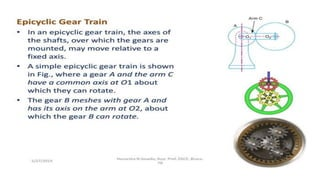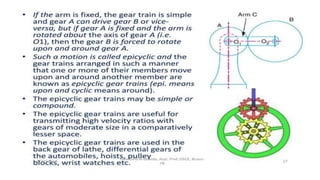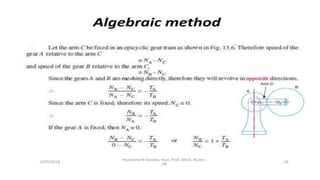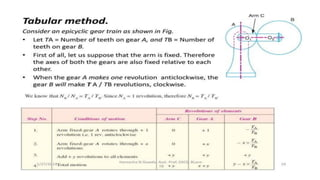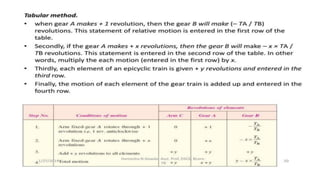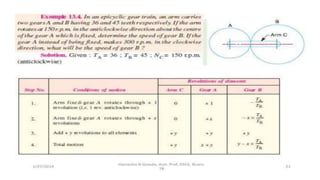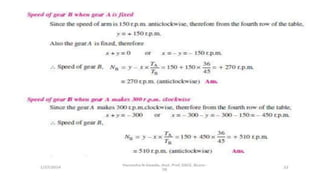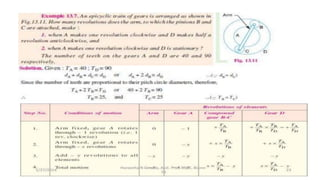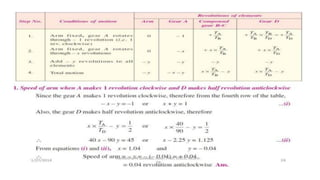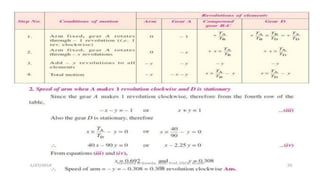1 von 30
Anzeige

### ch 4 gear trains.pdf

1. Gear Trains • A gear train is a combination of several gears arranged in a chain fashion such that the follower of one combination is the driver of the next, and so on. • In gear trains, therefore, some are drivers and others are followers. • Gear trains are widely used in many kinds of mechanism whenever a change in speed or torque of rotating members is required. Angular velocity ratio • Angular velocity ratio is the ratio of the output angular velocity to the input angular velocity and it is designated by e. 𝑒 = 𝜔𝑓𝑜𝑙𝑙𝑜𝑤𝑒𝑟 𝜔𝑑𝑟𝑖𝑣𝑒𝑟 = 𝜔𝑜𝑢𝑡𝑝𝑢𝑡 𝜔𝑖𝑛𝑝𝑢𝑡
2. • When gears are combined to form gear trains, it is necessary to employ positive and negative signs for speed to indicate whether the speed is in the same or opposite sense from a preselected direction. • Usually, the sign is placed by inspection. • In a simple gear train in many drive applications, an idler gear is used to bridge over the space between the driver and follower. • This idler gear does not effect the velocity ratio of the system. • The velocity ratio e in a gear train is determined in terms of the number of teeth of the drivers and followers in the chain. • For the external gear train, ignoring the sense of rotation, the angular velocity ratio is 𝑒 = 𝜔𝑓𝑜𝑙𝑙𝑜𝑤𝑒𝑟 𝜔𝑑𝑟𝑖𝑣𝑒𝑟
3. • The Diametral pitch of a pair of gears in mesh is defined as 𝑃 = 𝑛𝑢𝑚𝑏𝑒𝑟 𝑜𝑓 𝑡𝑒𝑒𝑡ℎ 𝑜𝑛 𝑡ℎ𝑒 𝑔𝑒𝑎𝑟 𝑝𝑖𝑡𝑐ℎ 𝑑𝑖𝑎𝑚𝑒𝑡𝑒𝑟 = 𝑁 𝑑 • Noting that the peripheral velocity at the point of contact to be 𝑉 = 𝜔2𝑑2 = 𝜔3𝑑3 The velocity can be determined in terms of the Diametral pitch 𝑉 = 𝜔2 𝑁2 𝑃 = 𝜔3 𝑁3 𝑃 Or, 𝜔3 𝜔2 = 𝑁2 𝑁3 Therefore, the velocity ratio is given by 𝑒 = 𝜔𝑜𝑢𝑡𝑝𝑢𝑡 𝜔𝑖𝑛𝑝𝑢𝑡 = − 𝜔3 𝜔2 = − 𝑁2 𝑁3 Where 𝑁2=number of teeth on the driver, and 𝑁3= number of teeth on the follower
4. Fig 4.1 Simple gear trains (a) external gear train (b) internal gear train • For the internal gear train shown in fig 4.1 (b), the velocity ratio is given by. 𝑒 = 𝜔𝑜𝑢𝑡𝑝𝑢𝑡 𝜔𝑖𝑛𝑝𝑢𝑡 = + 𝜔3 𝜔2 = + 𝑁2 𝑁3
5. • Noting that the velocity ratio is given by the ratio of the number of teeth on the driver and driven gears, we can write that 𝑒 = 𝜔𝑜𝑢𝑡𝑝𝑢𝑡 𝜔𝑖𝑛𝑝𝑢𝑡 = 𝑛𝑢𝑚𝑏𝑒𝑟 𝑜𝑓 𝑡𝑒𝑒𝑡ℎ 𝑜𝑛 𝑡ℎ𝑒 𝑑𝑟𝑖𝑣𝑒𝑟 𝑛𝑢𝑚𝑏𝑒𝑟 𝑜𝑓 𝑡𝑒𝑒𝑡ℎ 𝑜𝑛 𝑡ℎ𝑒 𝑓𝑜𝑙𝑙𝑜𝑤𝑒𝑟 = 𝑁2 𝑁3 Where the sign is to be placed by inspection. In general, for a gear train where some gears are drivers and others are followers, the velocity ratio is given by 𝑒 = 𝜔𝑜𝑢𝑡𝑝𝑢𝑡 𝜔𝑖𝑛𝑝𝑢𝑡 𝑒 = 𝑝𝑟𝑜𝑑𝑢𝑐𝑡 𝑜𝑓 𝑡𝑒𝑒𝑡ℎ 𝑛𝑢𝑚𝑏𝑒𝑟𝑠 𝑜𝑓 𝑡𝑒𝑒𝑡ℎ 𝑜𝑛 𝑡ℎ𝑒 𝑑𝑟𝑖𝑣𝑒𝑟 𝑝𝑟𝑜𝑑𝑢𝑐𝑡 𝑜𝑓 𝑡𝑒𝑒𝑡ℎ 𝑛𝑢𝑚𝑏𝑒𝑟𝑠 𝑜𝑓 𝑡𝑒𝑒𝑡ℎ 𝑜𝑛 𝑡ℎ𝑒 𝑓𝑜𝑙𝑙𝑜𝑤𝑒𝑟
6. • For the gear train in fig 4.2, the velocity ratio is found as follows Fig 4.2 Compound gear train
7. 𝑒1 = 𝜔3 𝜔2 = 𝑁2 𝑁3 𝑒2 = 𝜔4 𝜔3 = 𝑁3 𝑁4 𝑒6 = 𝜔6 𝜔5 = 𝑁5 𝑁6 For the gear train the velocity ratio is 𝑒 = 𝑒1𝑒2𝑒6 Which, in terms of the teeth numbers, is obtained to be 𝑒 = 𝑁2 𝑁4 𝑁5 𝑁6
8. • From the above velocity ratio equation, it can be noted that: i) The idler gear 3 does not affect the velocity ratio, and ii) The velocity ratio is given by 𝑒 = 𝑝𝑟𝑜𝑑𝑢𝑐𝑡 𝑜𝑓 𝑡𝑒𝑒𝑡ℎ 𝑛𝑢𝑚𝑏𝑒𝑟𝑠 𝑜𝑓 𝑡𝑒𝑒𝑡ℎ 𝑜𝑛 𝑡ℎ𝑒 𝑑𝑟𝑖𝑣𝑒𝑟 𝑝𝑟𝑜𝑑𝑢𝑐𝑡 𝑜𝑓 𝑡𝑒𝑒𝑡ℎ 𝑛𝑢𝑚𝑏𝑒𝑟𝑠 𝑜𝑓 𝑡𝑒𝑒𝑡ℎ 𝑜𝑛 𝑡ℎ𝑒 𝑓𝑜𝑙𝑙𝑜𝑤𝑒𝑟 In analyzing gear trains, it is convenient to express angular speeds in revolutions per minute , rpm.
Anzeige